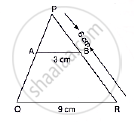Share

Books Shortlist
Your shortlist is empty

# Solution for In the Following Figure, Ab || Qr. Find the Length of Pb. - CBSE Class 10 - Mathematics

ConceptCriteria for Similarity of Triangles

#### Question

In the following figure, AB || QR. Find the length of PB.#### Solution

We have, ΔPAB and ΔPQR

∠P = ∠P [common]

∠PAB = ∠PQR [corresponding angles]

Then, ΔPAB ~ ΔPQR [By AA similarity]

therefore"PB"/"PR"="AB"/"QR"      [Corresponding parts of similar Δare proportional]

rArr"PB"/6=3/9

rArr"PB"=3/9xx6=2 cm

Is there an error in this question or solution?

#### Video TutorialsVIEW ALL 

Solution for question: In the Following Figure, Ab || Qr. Find the Length of Pb. concept: Criteria for Similarity of Triangles. For the course CBSE
S